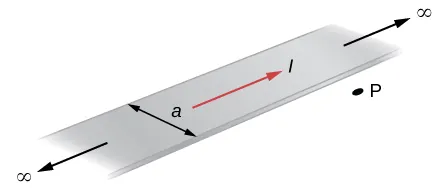University Physics Volume 2

# Challenge Problems

University Physics Volume 2Challenge Problems

### Challenge Problems

89.

The accompanying figure shows a flat, infinitely long sheet of width a that carries a current I uniformly distributed across it. Find the magnetic field at the point P, which is in the plane of the sheet and at a distance x from one edge. Test your result for the limit $a→0.a→0.$90.

A hypothetical current flowing in the z-direction creates the field $B→=C[(x/y2)i^+(1/y)j^]B→=C[(x/y2)i^+(1/y)j^]$ in the rectangular region of the xy-plane shown in the accompanying figure. Use Ampère’s law to find the current through the rectangle.91.

A nonconducting hard rubber circular disk of radius R is painted with a uniform surface charge density $σ.σ.$ It is rotated about its axis with angular speed $ω.ω.$ (a) Find the magnetic field produced at a point on the axis a distance h meters from the center of the disk. (b) Find the numerical value of magnitude of the magnetic field when $σ=1C/m2,σ=1C/m2,$ $R=20 cm,h=2 cm,R=20 cm,h=2 cm,$ and $ω=400rad/sec,ω=400rad/sec,$ and compare it with the magnitude of magnetic field of Earth, which is about 1/2 Gauss.

Order a print copy

As an Amazon Associate we earn from qualifying purchases.